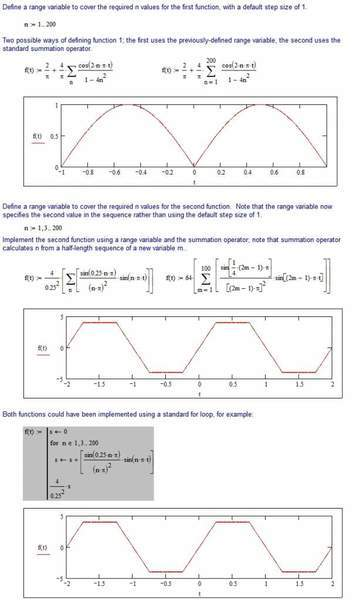# Plotting series in Matlab

• MATLAB
I am going to plot two series in Matlab. They are as following. please help me to write the appropriate code for them:

1-f(t)=(2/pi)+(4/pi)*Ʃ(1/(1-4*n^2))*cos(2*n*pi*t) -1≤t≤1 n=1 forN=200

2-f(t)=(4/0.25^2)*Ʃ(sin(0.25*n*pi)/(n*pi)^2)*sin(n*pi*t) -2≤t≤2 n=1,3,5 forN=200

Simon Bridge
Homework Helper
Welcome to PF:
You want to construct a vector for n, the use that and your formula to compute a vector of each element in the series.

The complication appears to be that you have two variables: n and t.
So you'll probably have to construct vectors for the different frequencies as well as the coefficients ... with the aim of ending up with a 2D matrix whose columns are the elements of the sum (corresponding to each n) and the rows are the functions at discrete samples (corresponding to values of t).

Summing the columns provides a row vector whose entries are f(t) sampled for discrete values of t. Then you can plot(t,f).

Could you please confirm the parentheses are correct in the two expressions? As written, I interpret the summation to be over n, ie {sum [1/(1-4*n^2)*cos(2*n*pi*t)] over n=1..200} but the expression 1 appears to be written as cos(2*n*pi*t)*{sum[1/(1-4*n^2)] over n=1..200} (et sim for 2).

Do you want f as a function of t and variable n or just as a function of t with the specified range for n?

Simon Bridge
Homework Helper
I ust figured everything after the sigma was included in the sum.

for 1.$$f(t)=\frac{2}{\pi} + \frac{4}{\pi} \sum_{n=1}^{200} \frac{\cos(2n\pi t)}{1-4n^2}$$... and for 2.$$f(t)=64\sum_{n=1}^{200} \frac{\sin(\frac{n\pi}{4})}{(n\pi)^2}\sin(n\pi t)$$

I still think the strategy I've outlined is the way to go here - we should avoid actually providing the code though.

Welcome to PF:
You want to construct a vector for n, the use that and your formula to compute a vector of each element in the series.

The complication appears to be that you have two variables: n and t.
So you'll probably have to construct vectors for the different frequencies as well as the coefficients ... with the aim of ending up with a 2D matrix whose columns are the elements of the sum (corresponding to each n) and the rows are the functions at discrete samples (corresponding to values of t).

Summing the columns provides a row vector whose entries are f(t) sampled for discrete values of t. Then you can plot(t,f).

That would be a possible solution.What if we do not consider sum command?

I ust figured everything after the sigma was included in the sum.

for 1.$$f(t)=\frac{2}{\pi} + \frac{4}{\pi} \sum_{n=1}^{200} \frac{\cos(2n\pi t)}{1-4n^2}$$... and for 2.$$f(t)=64\sum_{n=1}^{200} \frac{\sin(\frac{n\pi}{4})}{(n\pi)^2}\sin(n\pi t)$$

I still think the strategy I've outlined is the way to go here - we should avoid actually providing the code though.

In the second one n=1,3,5. everything else is right.

Well, here's how it might be done in Mathcad.

I have gut feeling (a colon sensation, one might say) that there is sum way of doing a similar thing in Matlab. I have a forboding that loops may occur to somebody, but they should probably be avoided.#### Attachments

Simon Bridge
Anyway = in matlab you need only do the step, so all the odd numbers are n=1:2:199; for all the odd numbers from 1 to 200. Writing it out in sigma notation is trickier than that... I need to sum over integer m from 0 to 99 and n=2m+1 and it looks like:$$f(t)=64\sum_{n=1}^{200} \sin\left ( \frac{(2n-1)\pi}{4}\right ) \frac{\sin((2n-1)\pi t)}{((2n-1)\pi)^2}$$... for the first 200 odd numbers.